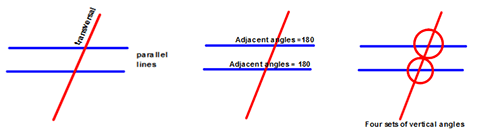# Angle Relationships (cont.)

Vertical angles, also known as opposite angles, are opposite angles formed by two intersecting lines. Vertical angles are ALWAYS congruent (angles that have the same measure) and tend to resemble bow ties.

Check it out! Look at the figure below where the lines intersect and the angles formed. You can see that the opposite angles are congruent.We can use what we have learned about supplementary angles and vertical angles to write and solve equations to find unknown angle measures. In the following figure, we are going to use the vertical angle relationship and the supplementary angle pair to find the measure of ∠ 1.• The given angle is vertical to ∠ 2 and is 54°. Remember that vertical angles are congruent. Therefore, the measure of ∠ 2 is 54°.
• Angles 1 and 2 are supplementary. That means the sum of their measures is 180°.
• We can write an equation now to find the measure of the unknown angle:
∠ 1 + 54 =180
-54     -54
∠ 1 = 126°

You saw a video previously that shows how to use vertical angles to find unknown angle measures.

There are other lines that can be used to help us find angles. We know that parallel lines are lines that lie in the same plane and never intersect. If you draw a line that intersects both of these parallel lines, you have created a transversal. The transversal creates eight angles through its intersection with the lines and if you know the measure of one of those angles, you can use what you have learned about adjacent and vertical angles to find the measures of the other seven angles.

Notice the figures below to get an idea of how this works.Watch this short video to see how transversals work with parallel lines to help you find angle measures of vertical and adjacent angles that form complementary and supplementary angles.

(source)